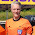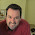## Tuesday, March 25, 2014

### 6 Ways for Students to Grapple with Definitions

This is a relatively short post. To prepare for workshops I am running for college math faculty this summer, I am thinking about the skills that an instructor needs to be able to be effective in using IBL. One of the big skills is building problem sets for students, and within that, one of the component skills is being able to help students develop an understanding of definitions.

Specifically, I am thinking about how I try to get students to begin processing the ideas in a definition, before putting their understanding to the test with problems or theorems that require application of the definition. What follows are 6 ways that I have thought of, so far, that get students to begin to attach meaning to a definition.

For purposes of illustration, I will refer to the definition of prime. A natural number p is prime if and only if p is not the product of natural numbers less than p.
1. Have students sort a list of candidates into examples and non-examples. Prime: Which of the numbers -3, -1, 1, 2, 3, 4, 5 are prime?
2. Fill in the blank with all, some, or none. Prime: All/some/none of the even natural numbers are prime. All/some/none of the odd natural numbers are prime.
3. Prove a very basic and concrete example. Prime: Prove that 3 is prime, using the definition. Prove that 4 is not prime, using the definition.
4. Ask students to construct their own examples. Prime: List 5 prime numbers. (Notice that I chose 5 here to force students to confront that 9 is odd, but not prime.)
5. Relate the current definition to concepts encountered earlier. Prime: We have been discussing divisibility in this course. Rewrite the definition of prime using forms of the word “divisibility” where appropriate.
6. Pose a true or false question about the definition. Prime: True or false: 2 is the only even number that is prime.
The main idea in all of these is to get students to unravel the definition by learning to distinguish the important features of the definition, especially which objects are included and which are excluded. I do not use all of these methods with every definition, but rather use a couple of them for each new term. Depending on students’ familiarity with the specific definition, asking students to construct their own examples is typically more difficult than the other tasks. Also, non-examples almost certainly must be supplied by the instructor, since a student, by definition just learning about the idea, is unlikely to have an idea of what kinds of objects are useful non-examples. For instance, a ballpoint pen is not a prime number, but this is not a useful non-example! However, note that students can be asked to determine whether a mathematical object is or is not an example of the definition being introduced.

I would love to add to this list. Readers, please share your suggestions!

#### 2 comments:

1.I like your list. I can only add that I sometimes take a very direct approach, and then your list naturally occurs to students as they work. One of my favorite parts of my Euclidean Geometry class is asking the students to give a concrete definition for each of the following terms: polygon; convex polygon; simple polygon; the interior of a polygon. It takes weeks of work. When we are done, they appreciate the care and feeding of good definitions much more.

1.Thanks TJ. Definition building is a good strategy.